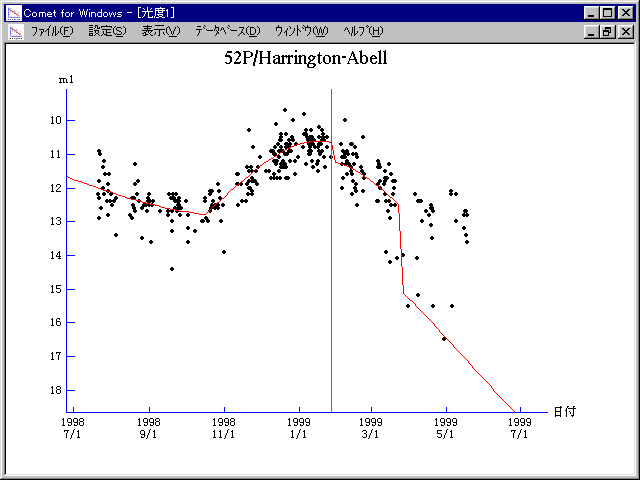# 52P/Harrington-Abell (1999)

 Japanese version Home page Updated on June 12, 1999###Pictures###Orbital Elements

```   The following improved orbital elements by Kenji Muraoka,
are from 318 observations 1975 to 1999, including 9 Planets,
Moon and 5 minor planets perturbations and non-gravitational
effect of style II.  The mean residual is +/- 0.71 arc seconds.

Epoch  =  1999 Jan  = 2451200.5
T  =  1999 Jan. 27.87697       +/- 0.00058 (m.e.) TT
Peri. =  138.90063                +/- 0.00025
Node  =  337.28741                +/- 0.00008   (2000.0)
Incl. =   10.21847                +/- 0.00001
q  =    1.7559831              +/- 0.0000015 AU
e  =    0.5429107              +/- 0.0000004
a  =    3.8416632              +/- 0.0000013 AU
n  =    0.13089563             +/- 0.00000006
P  =    7.530                  +/- 0.0000037  years
A1  =   +0.320                  +/- 0.021
A2  =   +0.00563                +/- 0.00039
```

###Finding Charts###Magnitudes Graph

```        m1 = 19.5 + 5 log d - 25   log r  [    ,-100]  (             - 1998 Oct. 19)
m1 =  7.4 + 5 log d + 15.0 log r  [-100,   0]  (1998 Oct. 19 - 1999 Jan. 27)
m1 =  5.5 + 5 log d + 25.0 log r  [   0,  55]  (1999 Jan. 27 - 1999 Mar. 23)
m1 =  8.0 + 5 log d + 25   log r  [  55,    ]  (1999 Mar. 23 -             )
```##### The orbital elements are calculated by Kenji Muraoka. The charts are made with StellaNavigator Ver.2.0 for Windows (AstroArts / ASCII). The magnitudes graphs are made with Comet for Windows.